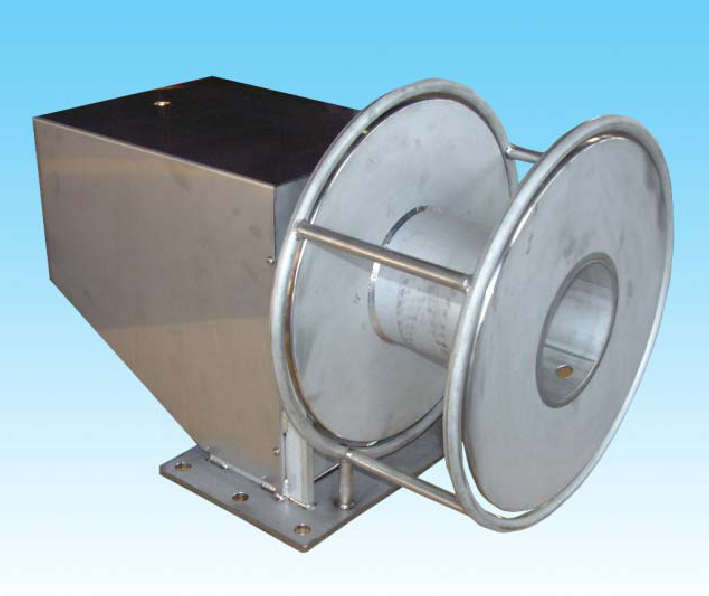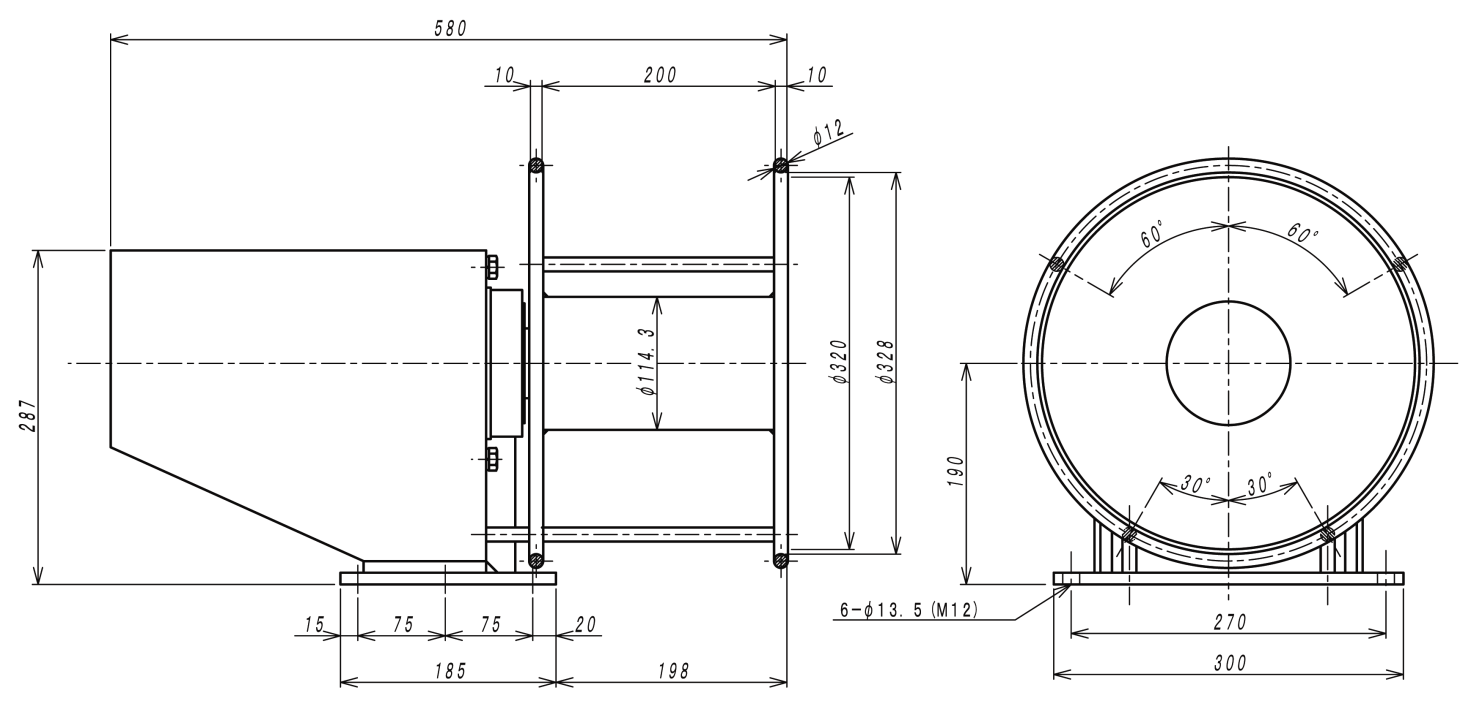# Hoist## Hoist

The hoist is made from SUS304 and the corrosion-resistance is extremely superior.

### Outer dimension### Specification

Ex) Model : GH261## I　Main calculation formula of hydraulic pressure device

1. ### Hydraulic pump

• 1)　axis input of a pump
Ls=(P･Q/60η)･102
• 2)　Oil power of the pump
Lp= P･Q/60＝η･Ls・10-2
• 3)　Total efficiency of the pump
η=ηv･ηt・10-2
• 4)　Capacity efficiency of the pump
ηv=(Q/Qth)･100≒(Q/Q0)･100
• 5)　Efficiency of the motor
ηe= Ls/ Le
2. ### Hydraulic motor

• 1)　Theoretical Displacement volume of Hydraulic motor
Dth=(2π･T)/(P･ηt)･102
• 2)　Output power of Hydraulic motor
Ls=2π･T･N/60000=η･(P･Q/60)･102
• 3)　Input power of Hydraulic motor
Lm=P･Q/60
• 4)　Capacity efficiency of Hydraulic motor
ηv= (Dth･N/Q)･10-1
• 5)　Torque efficiency of Hydraulic motor
ηt= (2π･T/P･Dth )･102
• 6)　Total efficiency of Hydraulic motor
η=ηv･ηt･102= (Ls/ Lm)･102=(2π･T･N/P･Q)･10-1
3. ### Cylinder

• 1)　Necessary pressure to a cylinder
P1=(1/ A1)･(F/ηc+P2･A2･102) ･10-2
• 2)　Necessary flow rate for a cylinder
Q= A1･v･10-1+QL
• 3)　Driving force of a cylinder
Acceleration ability
F1=m･α=m･v1/t
Static wear resistance
F2= μs･m･g
Dynamic wear resistance
F3= μd･m･g

### The explanations of symbols

Ls: input of pump shaft, output power of the motor, the output power of the motor (kW)
Lp: oil power of the pump (kW)
Le: input power of the motor (kW)
Lm: input power of the motor (kW)
P: Discharge pressure of pump, differential-pressure of the input/output port of a motor (MPa)
P1: necessary pressure to cylinder (MPa)
P2: pressure of the cylinder inflow (MPa)
Q: discharge amount at the time of discharge pressure P,
inflow oil amount to the motor, necessary flow rate of the cylinder (large/min)
Qth: theoretical discharge amount (l/min)
Qo: discharge amount at the time of discharge pressure P≒0, (l/min)
QL: Leak inside the cylinder (l/min)
T: shaft torque (N・m)
N: 　number of rotations (min-1)
η: Total efficiency of the pump, total efficiency of the motor (the %)
η v: capacity efficiency of pump, capacity efficiency of the motor (the %)
η t: torque efficiency of pump, torque efficiency of the motor (the %)
η e: efficiency of motor (the %)
η c: driving force efficiency of cylinder (0.9-0.95)
Dth: theoretical displacement volume of the motor (cm3/rev)
A1: inlet side pressure receiving area of cylinder (cm2)
A2: outlet side pressure receiving area of cylinder(cm2)
F: cylinder driving force (N)
F1: cylinder acceleration force (N)
F2: static wear resistance (N)
F3: dynamic wear resistance (N)
v: The speed of the cylinder (m/min)
v1: The speed after acceleration (m/s)
m: The mass of the load (KG)
α: acceleration (m/s2)
t: acceleration time (s)
μ s: static friction coefficient
μ d: dynamic friction coefficient
g: acceleration of the gravity (m/s2)